# Class 12 Board Exams - Important Topics In Physics You Must Never Omit

###### ByRamraj Saini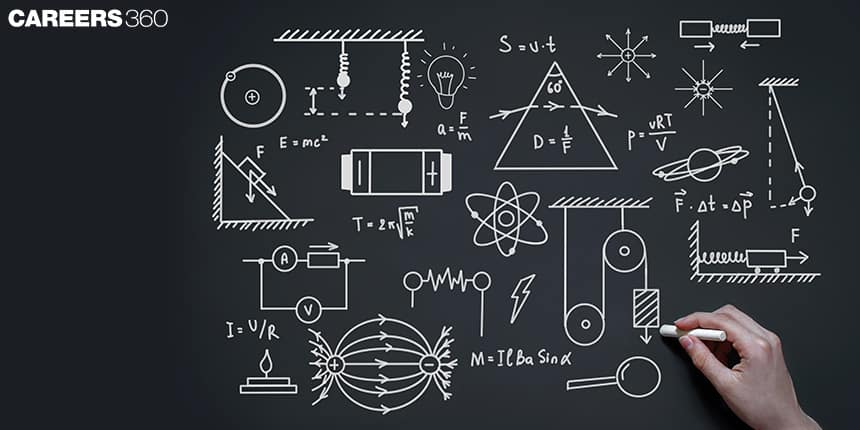###### Synopsis

Each subject has equal importance in board examinations but all subjects do not have the same difficulty level, some of them are easy and some are difficult. Physics is considered as one of the toughest subjects in CBSE as well as State Boards examinations across the country. Many students do not score well in it. Careers360 has prepared a unit-wise weightage distribution for physics that will guide you to study those topics first which have the most weightage and thus, improve your score. So start your prep right away!###### Synopsis

Each subject has equal importance in board examinations but all subjects do not have the same difficulty level, some of them are easy and some are difficult. Physics is considered as one of the toughest subjects in CBSE as well as State Boards examinations across the country. Many students do not score well in it. Careers360 has prepared a unit-wise weightage distribution for physics that will guide you to study those topics first which have the most weightage and thus, improve your score. So start your prep right away!

Class 12 Board Exams - Important Topics In Physics You Must Never Omit - Physics is an interesting and exciting subject to study due to its practical usefulness in real-world situations. From an exam point of view all the subjects are important for CBSE class 12 but Physics is considered to be a tough one because of its difficulty level. So, to excel in the subject you should be aware of the unit-wise weightage distribution. It includes the typology of questions that have been asked in the previous examinations or to be asked in upcoming examinations. Weightage distribution will help to decide how much time you should spend on a particular unit.

The CBSE class 12 Physics board exam will most likely be held first. So, the primary concern of the students is finishing up last-minute revisions and making the most of their study time. If you are not familiar with the unit-wise weightage distribution, read this article carefully.

## Weightage of Units of Different Topics

The comprehensive details about important topics and derivations are given below, that may be asked in the board examinations. Before moving to the important chapters, let us check the unit-wise weightage distribution for better understanding.There are two sections in the physics board examinations one is theory section and another one is practical (internal assessment).

• The marks distribution for the theory paper of CBSE Class 12 Boards according to units and chapters are given below.

 Unit/Topic Unit/Topic Name Weightage Unit 1 Electrostatics 10 Marks 1. Electric Charges and Fields 2. Electrostatic Potential and Capacitance Unit 2 Current Electricity 6 Marks 1. Current Electricity Unit 3 Magnetic Effects of Current and Magnetism 8 Marks 1. Moving Charges and Magnetism 2. Magnetism and Matter Unit 4 Electromagnetic Induction and Alternating Currents 9 Marks 1. Electromagnetic Induction 2. Alternating Current Unit 5 Electromagnetic Waves 2 Marks 1. Electromagnetic Waves Unit 6 Optics 16 Marks 1. Ray Optics and Optical Instruments 2. Wave Optics Unit 7 Dual Nature of Radiation and Matter 4 Marks 1. Dual Nature of Radiation and Matter Unit 8 Atoms and Nuclei 8 Marks 1. Atoms 2. Nuclei Unit 9 Electronic Devices 7 Marks 1. Semiconductor Electronics: Materials, Devices and Simple Circuits Total 70 Marks
• The practicals or internal assessment of CBSE board exams carries a weightage of 30 marks, which are divided into four parts, as follows,
 Topics Marks Two experiments one from each section 16 Marks (8 + 8) Practical Record 7 Marks Viva on experiments 7 Marks Total 30 Marks

As we have seen in the above table Optics, Electrostatics, Electromagnetic Induction and Alternating Currents, Magnetic Effects of Current and Magnetism, Atoms and Nuclei and Electronic Devices have more weightage than units. So make sure you focus more on these units to get desired marks in the examination.

We know that every topic has two types of questions, theoretical questions (derivations and some short answer questions) and numerical questions, these are based on formulas. So, plan accordingly what type of questions you can deal with in a good manner. Here we will discuss important topics in order to weigh them in board examinations.

## UNIT 6: Optics (16 marks)

Optics has the most weightage in theory section as well as in practical section. This unit is further divided into two chapters, Ray Optics and Optical Instruments and Wave Optics. Almost every time in the board examination one of the derivations and two or three numericals are asked from this unit. Some of the most important topics covered in this chapter are given below:

Chapter 9 : Ray Optics and Optical Instruments

• Image formation by spherical mirrors and lenses.(Numericals)

• Combination of lenses and power.(Numericals)

• Total internal reflection (TIR).

• Natural phenomenon due to light (Definition and Numericals)

• Refraction through a Prism (Numericals, Derivations and Ray diagram)

• Eye with different diseases

• Microscope and Telescope(Derivation)

Chapter 10 : Wave optics

• Waves addition ( Incoherent and coherent )

• Refraction and reflection of plane waves (using Huygen principle)

• Diffraction of light

• Interference and Young’s double-slit experiment

• Polarisation ( By scattering Resolving power of optical Instruments)

## UNIT 1: Electrostatics (10 Marks)

Electrostatics has the second highest weightage in physics board examinations. This unit is further divided into two chapters, Electric Charges and Fields and Electrostatic Potential and Capacitance. There is more probability to ask for derivations from this unit because there are many derivations in the unit. Key topics and derivations of this unit are listed below:

Chapter 1 : Electric Charges and Field

• Coulomb’s Law (Numericals)

• Forces between multiple charges electric field due to system of charges

• Electric dipole in the external electric field

• Electric dipole’s electric field on the axial and equatorial point

• Gauss’s law and its applications

Chapter 2 : Electrostatic Potential and Capacitance

• Equipotential surfaces

• Potential due to electric dipole and system of charges

• Electric potential energy due to electric dipole and system of charges

• Effect of dielectric on capacitors

• Energy stored in capacitors

• Combinations of capacitors

## UNIT 4: Electromagnetic Induction and Alternating Current (9 Marks)

This unit has the third highest weightage in physics board examinations. This unit is further divided into two chapters, Electromagnetic Induction & Alternating Current. From this unit both types of the questions have been asked for derivations as well as numericals. So, plan accordingly. Some important key topics and derivations of this unit are given below:

Chapter 6: Electromagnetic Induction

• Lenz’s law

• Magnetic flux

• Motional EMF

• Self-inductance and mutual inductance

Chapter 7: Alternating Current

• RC, LC, LR and LCR Circuits (derivations and Numericals)

• LC oscillations (Derivations and Numericals)

• Transformers

• Power factor

• Resonance and sharpness

Also check - Know All About The Mettl Employability Test

## UNIT 3: Magnetic Effects of Current and Magnetism (8 Marks)

This unit is also further divided into two chapters, Moving Charges and Magnetism and Magnetism and Matter. There is more probability to ask numerical from this unit. Some important key topics and derivations of these chapters are given below:

Chapter 4: Moving Charges and Magnetism

• Motion in a magnetic field

• Magnetic force on current-carrying wire with theory and derivation.

• Magnetic-field on the axis of the circular current-carrying loop

• Cyclotron (Theory, Diagram and Derivation)

• Velocity selector

• The force between two parallel current-carrying wires

• Ampere’s circuital law

• Torque on current-carrying loop in a magnetic field

• Moving coil galvanometer

Chapter 5: Magnetism and Matter

• Electric dipole in a uniform magnetic field

• The axial magnetic field of a bar magnet as solenoid

• Hysteresis Loop

• Diamagnetic, Paramagnetic, Ferromagnetic Substances

## UNIT 8: Atoms and Nuclei (8 Marks)

This unit is further divided into two chapters, Atoms and Nuclei. From this unit most of the time numericals have been asked so you can focus more on numerical for this unit. Some important key topics and derivations of these chapter are listed below:
Chapter : 12 – Atoms

• Atomic spectrum

• Electron Orbits

• de-Broglie explanation of Bohr’s postulate

• Bohr Model of a Hydrogen atom

• Line spectra of a Hydrogen atom

Chapter 13: Nuclei

• Nuclear binding energy (Definition and Numericals)

## UNIT 9: Electronic Devices (7 Marks)

This unit includes only one chapter, Semiconductor Electronics: Materials, Devices, and Simple Circuits. Most of the questions asked in examinations from this chapter are from diode and logic gates. These are some of the most important topics covered in this chapter:

Chapter 14: Semiconductor Electronics: Materials, Devices and Simple Circuits

• Semiconductors ( Intrinsic and Extrinsic )

• p-n Junction diode ( reverse bias and forward bias )

• Transistor ( n-p-n and p-n-p , As an amplifier )

• Junction diode ( As a rectifier )

• Zener diode (As a voltage regulator )

• Logic gates

Unit 2 ( Current Electricity ), Unit 5 ( Electromagnetic Waves) and Unit 7 ( Dual nature of radiation and Matter ) contain more topics having less weightage ( only 12 marks together which is less than only optics ) in Class 12 Boards. So, according to the marks distribution you can focus more on high weightage units so that you can get optimum marks in the examinations.

• Physical sciences
• Physics
• Electricity
• Electromagnetism
• Magnetic field
• Magnetism
• Optics
• Physical quantities
• Electrical engineering
• Diode

## Careers360 helping shape your Career for a better tomorrow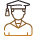#### 250M+

Students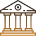#### 30,000+

Colleges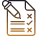#### 500+

Exams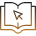E-Books